# 模型解释–SHAP Value的简单介绍2020年6月7日07:07:00311 9780字阅读32分36秒

## SHAP Value方法的介绍

SHAP的目标就是通过计算x中每一个特征对prediction的贡献, 来对模型判断结果的解释. SHAP方法的整个框架图如下所示：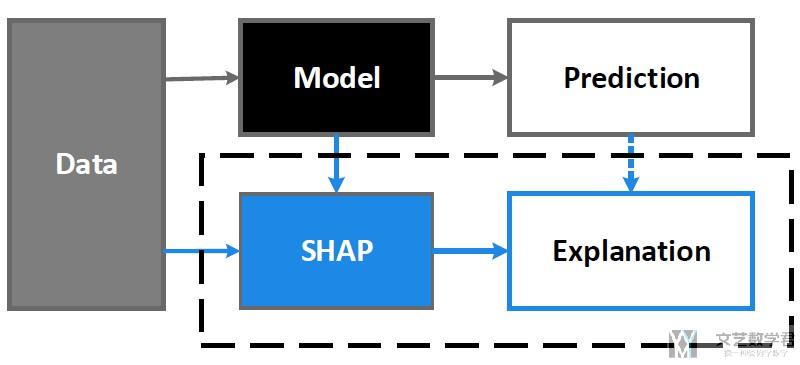SHAP Value的创新点是将Shapley Value和LIME两种方法的观点结合起来了. One innovation that SHAP brings to the table is that the Shapley value explanation is represented as an additive feature attribution method, a linear model. That view connects LIME and Shapley Values.

SHAP解释的时候使用下面的表达式, 这个和LIME中的原理是相似的(最大的不同是SHAP中设置了不同的distance的定义, 我们后面会讲):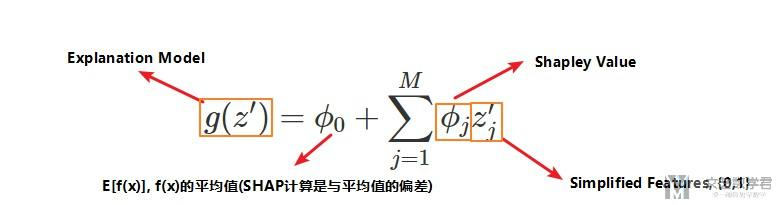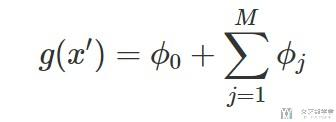### KernelSHAP的简单介绍

KernelSHAP包含下面的5个步骤:

1. 初始化一些数据, z', 作为Simplified Features, 例如随机生成(0, 1, 0, 1), (1, 1, 1, 0)等.
2. 将上面的Simplified Features转换到原始数据空间, 并计算对应的预测值, f(h(z')).
3. 对每一个z'计算对应的权重(这里权重的计算是关键, 也是SHAP与LIME不同的地方)
4. 拟合线性模型
5. 计算出每一个特征的Shapley Value, 也就是线性模型的系数.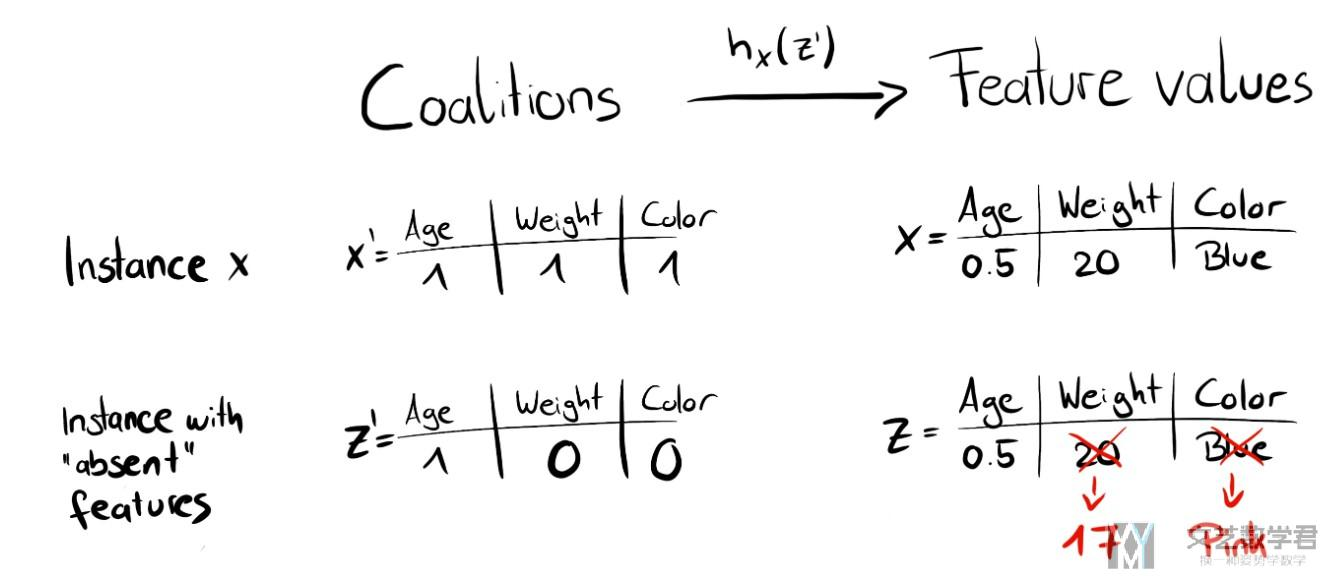LIME根据相似度进行计算距离, 如sample的数据中有很多0, 也就是会和原始数据有很大的不同, 那么他的距离是远的, 也就是他的权重会是小的. (LIME weights the instances according to how close they are to the original instance. The more 0's in the coalition vector, the smaller the weight in LIME.)

SHAP中距离的计算根据Simplified Features中0的数量, 若有很多0或是很多1, 他的权重都是比较高的.

• 这是因为若都是0, 只有一个是1, 那么我们可以很好的计算出那个是1的特征的贡献.
• 若只有一个是0, 我们可以计算出那个是0的特征的贡献.
• 如果一半是0, 一半是1, 那么会有很多种组合, 就很难计算出每一个特征的贡献(下面是原文).

SHAP weights the sampled instances according to the weight the coalition would get in the Shapley value estimation. Small coalitions (few 1's) and large coalitions (i.e. many 1's) get the largest weights. The intuition behind it is: We learn most about individual features if we can study their effects in isolation.

• If a coalition consists of a single feature, we can learn about the features' isolated main effect on the prediction.
• If a coalition consists of all but one feature, we can learn about this features' total effect (main effect plus feature interactions).
• If a coalition consists of half the features, we learn little about an individual features contribution, as there are many possible coalitions with half of the features.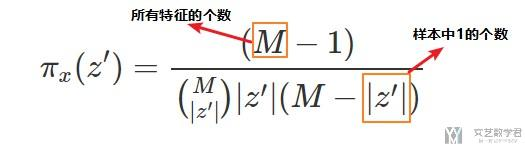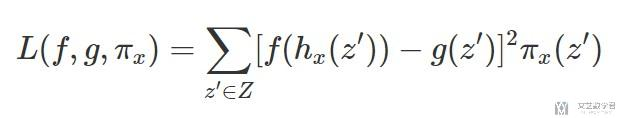## SHAP的简单使用方法

SHAP provides multiple explainers for different kind of models.

• TreeExplainer : Support XGBoost, LightGBM, CatBoost and scikit-learn models by Tree SHAP.
• DeepExplainer (DEEP SHAP) : Support TensorFlow and Keras models by using DeepLIFT and Shapley values.
• GradientExplainer : Support TensorFlow and Keras models.
• KernelExplainer (Kernel SHAP) : Applying to any models by using LIME and Shapley values.

SHAP的帮助文档 : SHAP (SHapley Additive exPlanations)

### 需要注意--出现报错的解决

1. class Net(nn.Module):
2.     def __init__(self):
3.         super(Net, self).__init__()
4.         self.conv_layers = nn.Sequential(
5.             nn.Conv2d(1, 10, kernel_size=5),
6.             nn.MaxPool2d(2),
7.             nn.ReLU(),
8.             nn.Conv2d(10, 20, kernel_size=5),
9.             nn.Dropout(),
10.             nn.MaxPool2d(2),
11.             nn.ReLU(),
12.         )
13.         self.fc_layers = nn.Sequential(
14.             nn.Linear(320, 50),
15.             nn.ReLU(),
16.             nn.Dropout(),
17.             nn.Linear(50, 10),
18.             nn.Softmax(dim=1)
19.         )
20.     def forward(self, x):
21.         x = self.conv_layers(x)
22.         x = x.view(-1, 320) # doesn't affect the gradient, so can be outside an nn.Module object
23.         x = self.fc_layers(x)
24.         return x

1. class Net(nn.Module):
2.     def __init__(self):
3.         super(Net, self).__init__()
4.         self.conv1 = nn.Conv2d(1, 10, kernel_size=5)
5.         self.conv2 = nn.Conv2d(10, 20, kernel_size=5)
6.         self.conv2_drop = nn.Dropout2d()
7.         self.fc1 = nn.Linear(320, 50)
8.         self.fc2 = nn.Linear(50, 10)
9.     def forward(self, x):
10.         x = F.relu(F.max_pool2d(self.conv1(x), 2))
11.         x = F.relu(F.max_pool2d(self.conv2_drop(self.conv2(x)), 2))
12.         x = x.view(-1, 320)
13.         x = F.relu(self.fc1(x))
14.         x = F.dropout(x, training=self.training)
15.         x = self.fc2(x)
16.         return F.softmax(x, dim=1)

### SHAP例子分析--Iris dataset

#### 准备工作

1. import shap
2. shap.initjs() # 用来显示的

#### 创建解释器

1. # 新建一个解释器
2. # 这里传入两个变量, 1. 模型; 2. 训练数据
3. explainer = shap.KernelExplainer(batch_predict, x)
4. print(explainer.expected_value) # 输出是三个类别概率的平均值
5. """
6. [0.32909563 0.33329453 0.33760984]
7. """

#### 对单个数据进行解释

1. # 选择一个数据进行解释(还是选择第5个数据)
2. shap_values = explainer.shap_values(x)

#### 解释结果可视化

1. # 对单个数据进行解释
2. shap.force_plot(base_value=explainer.expected_value,
3.                 shap_values=shap_values,
4.                 feature_names=['sepal length','sepal width','petal length','petal width'],
5.                features=x)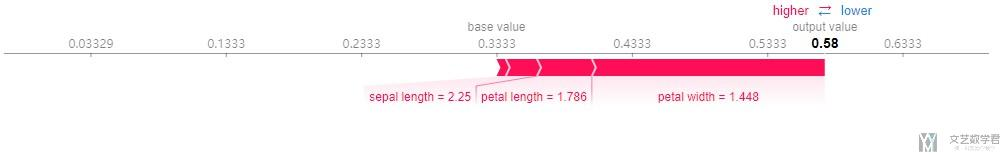1. shap.force_plot(base_value=0.5,
2.                 shap_values=np.array([0.1, 0.2, 0.3, -0.1]),
3.                 feature_names=['sepal length','sepal width','petal length','petal width'],
4.                features=np.array([0.1, 0.2, 0.3, 0.1]),
5.                out_names='VPN',
6.                text_rotation=30,
7.                matplotlib=True)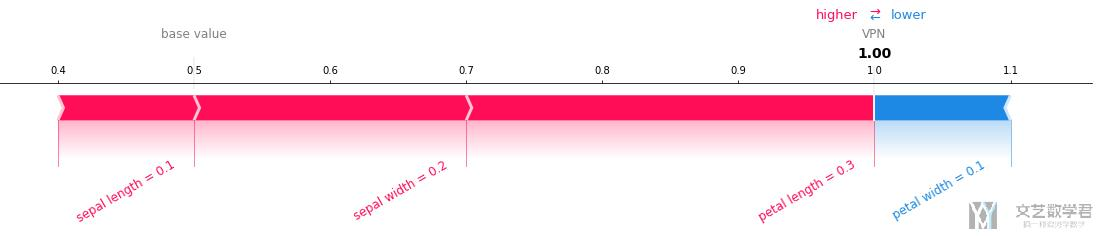#### 对特征重要度进行解释

1. # 对特征重要度进行解释
2. shap_values = explainer.shap_values(x)
3. # --------
4. # 进行绘图
5. # --------
6. shap.summary_plot(shap_values=shap_values,
7.                  features=x,
8.                  feature_names=['sepal length','sepal width','petal length','petal width'],
9.                  plot_type='bar')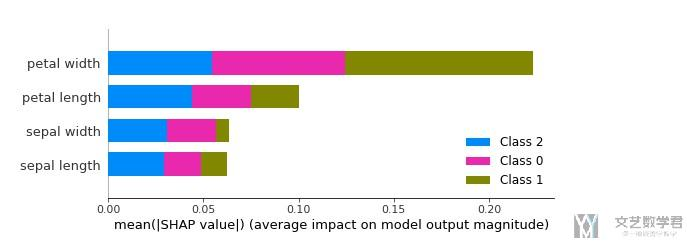### SHAP例子分析--每一类分析

#### 对单个数据的解释

1. # 新建一个解释器
2. X_exp = torch.from_numpy(XData).float()
3. explainer = shap.DeepExplainer(fullModel.to(device), X_exp.to(device))
4. print(explainer.expected_value)

1. # 随机选择1个数据, 来进行解释
2. X_exp = XData[-3]
3. X_tensor = torch.from_numpy(X_exp).unsqueeze(0).float().to(device)
4. # 计算样本的shape value
5. shap_values = explainer.shap_values(X_tensor)

1. # 得到特征的名字和解释数据的特征的值
2. feature_names=list(dfDataTrain.columns[:-4])
3. features = features = np.around(X_exp,2) # 解释的数据的特征的值
4. # 对单个数据进行解释
5. t = shap.force_plot(base_value=explainer.expected_value[YData[-3]],
6.                 shap_values=shap_values[YData[-3]],
7.                 feature_names=feature_names,
8.                 features=features,
9.                 out_names = 'DoS Attack',
10.                 text_rotation=30,
11.                 matplotlib=True,
12.                 show=False)

1. t.set_facecolor('white') # 把背景颜色设置为白色
2. t.savefig('filename.png', bbox_inches = 'tight', dpi=500) # 保存图片#### 对特征重要度的解释

SHAP可以用来进行模型的整体解释, 我们只需要将训练集中所有数据的每一个特征都计算一遍, 计算平均值即可. 这里我们绘制出对每一个特征重要度的解释.

1. # 随机选择1个数据, 来进行解释
2. X_exp = XExplainDate
3. X_tensor = torch.from_numpy(X_exp).float().to(device)
4. # 计算样本的shape value
5. shap_values = explainer.shap_values(X_tensor)
6. # ---------
7. # 进行绘图
8. # ---------
9. plt.figure(dpi=500) # 设置图片的清晰度
10. fig = plt.gcf() # 获取后面图像的句柄
11. shap.summary_plot(shap_values=shap_values,
12.                   features = XData, # 所有样本的feature的值
13.                   feature_names=list(dfDataTrain.columns[:-4]), # 特征的名字
14.                  plot_type = 'bar',
15.                  max_display=20,
16.                  show=False)

1. fig.set_facecolor('white')
2. fig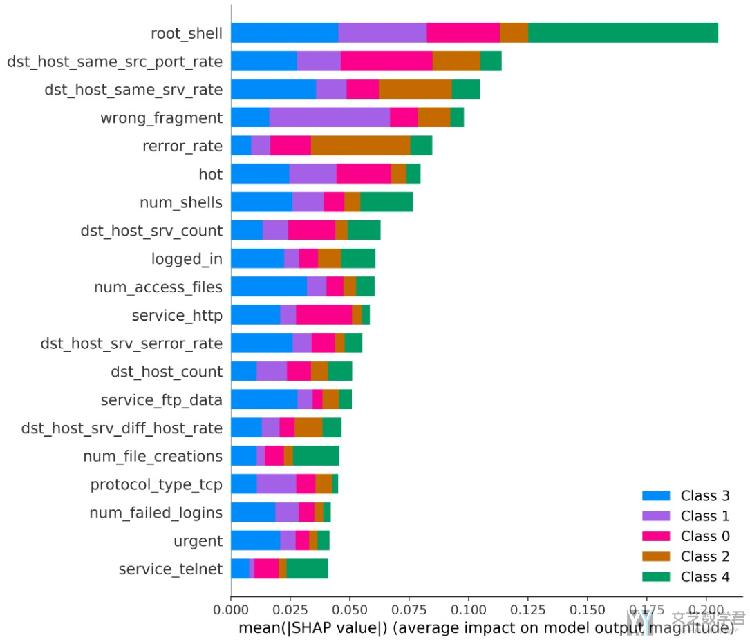1. shap.summary_plot(shap_values=shap_values,
2.                   features = XData, # 所有样本的feature的值
3.                   feature_names=list(dfDataTrain.columns[:-4]), # 特征的名字
4.                  plot_type = 'bar',
5.                  max_display=20,
6.                  show=False)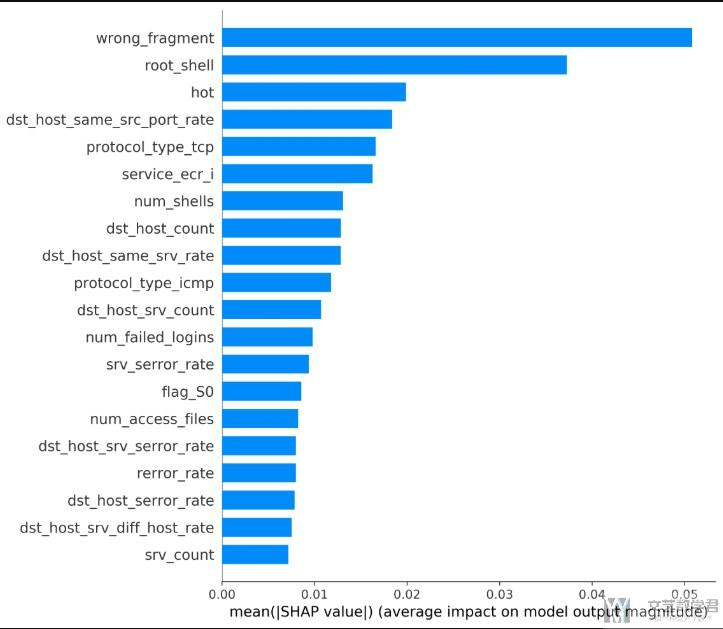#### 特征取值与重要度之间的关系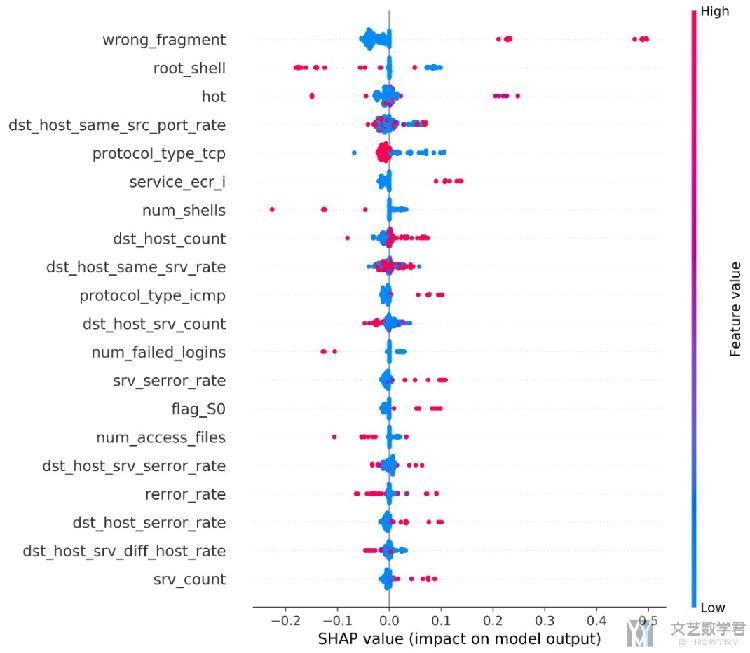1. shap.dependence_plot(ind = 'wrong_fragment',
2.                      shap_values=shap_values,
3.                      features = XExplainDate, # 所有样本的feature的值
4.                      feature_names=list(dfDataTrain.columns[:-4]), # 特征的名字
5.                      interaction_index = None,
6.                     )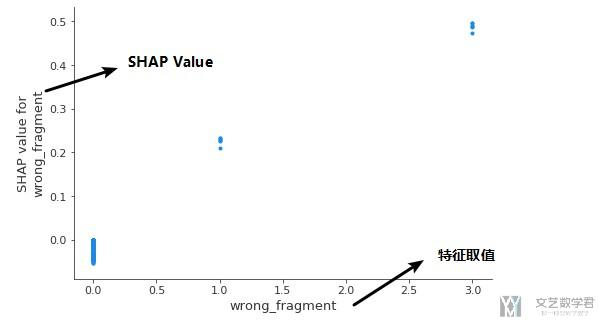#### 保存图像的方式

1. plt.figure(dpi=1200) # 设置图片的清晰度
2. fig = plt.gcf() # 获取后面图像的句柄
3. shap.summary_plot(X, show=False)
4. fig.set_facecolor('white') # 设置背景为白色

• 微信公众号
• 关注微信公众号
•• QQ群
• 我们的QQ群号
•• 本文由 发表于 2020年6月7日07:07:00
• 转载请务必保留本文链接：https://mathpretty.com/10699.html

•wwy

请问有完整代码吗

•wwy

请问有完整代码吗，可以学习一下吗

•王 茂南

@ wwy 你好，你可以参考一下这个链接，https://github.com/wmn7/ML_Practice/tree/master/2019_07_08/SHAP